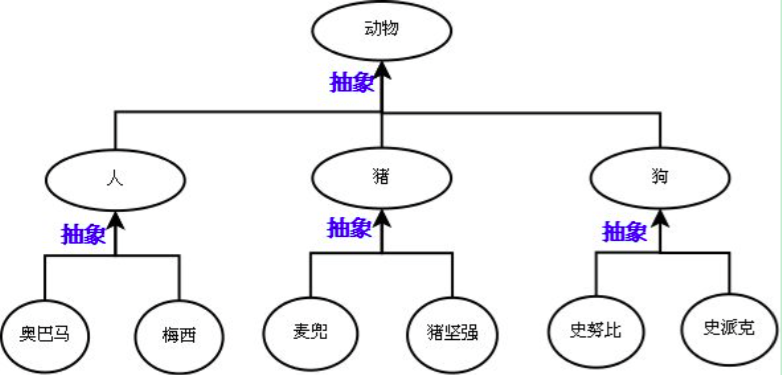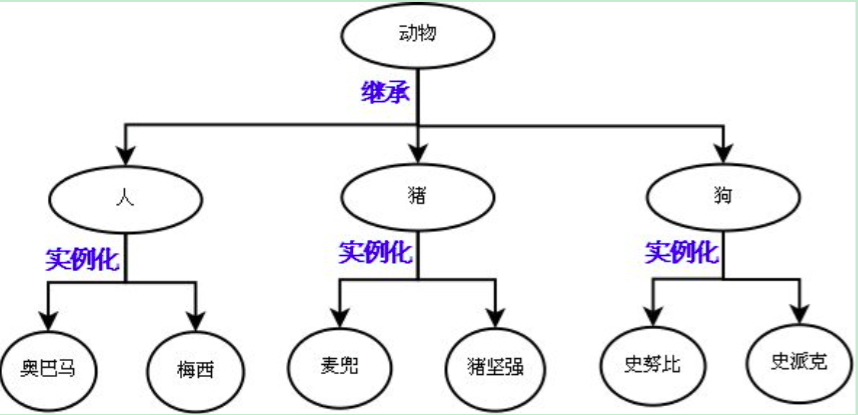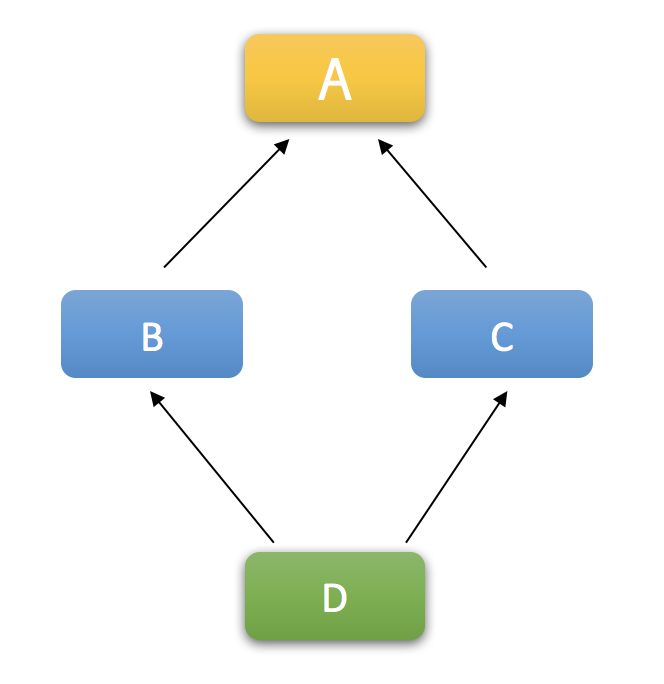# 面向对象进阶

## 类型判断

### issubclass

class Base:
pass

class Foo(Base):
pass

class Bar(Foo):
pass

print(issubclass(Bar, Foo))  # True
print(issubclass(Foo, Bar))  # False
print(issubclass(Bar, Base))  # True

### type

class Foo:
pass

obj = Foo()
print(obj, type(obj))  # 查看obj的类

### isinstance

isinstance也可以判断x是y类型的数据。

class Base:
pass

class Foo(Base):
pass

class Bar(Foo):
pass

print(isinstance(Foo(), Foo))  # True
print(isinstance(Foo(), Base))  # True
print(isinstance(Foo(), Bar))   # False

isinstance可以判断该对象是否是家族体系中的(只能往上判断类)。

## 反射

### 为什么需要反射？

def chi():
print("⼤⽜一顿吃100个螃蟹")

def he():
print("⼤牛一顿喝100瓶可乐")

def la():
print("⼤牛不⽤拉")

def shui():
print("⼤牛⼀次睡一年")

while 1:
print('''
作为大牛，我帮你写了
chi
he
la
shui
等功能，你自己看着办吧...
''')
func = input('请输入你要测试的功能：').strip()
if func == 'chi':
daniu.chi()
elif func == 'he':
daniu.he()
elif func == 'la':
daniu.la()
elif func == 'shui':
daniu.shui()
else:
print('大牛就这几个功能，别搞事情！')

### 什么是反射？

import daniu
while 1:
print('''
作为大牛，我帮你写了
chi
he
la
shui
等功能，你自己看着办吧...
''')
func_str = input('请输入你要测试的功能：').strip()
if hasattr(daniu, func_str):
func = getattr(daniu, func_str)
func()
else:
print('大牛就这几个功能，别搞事情！')

hasattr(对象, 字符串)是用来判断对象是否有字符串名称对应的这个属性(功能)。

getattr(对象，字符串)是用来获取对象中字符串名称对应的属性(功能)。

### 反射应用

class Person:
country = "China"

def eat(self):
pass

# 类中的内容可以这样动态的进⾏获取
print(getattr(Person, "country"))
print(getattr(Person, "eat"))  # 相当于Foo.func 函数
# 对象⼀样可以
obj = Person()
print(getattr(obj, "country"))
print(getattr(obj, "eat"))  # 相当于obj.func ⽅法

getattr可以从模块中获取内容，也可以从类中获取内容，也可以从对象中获取内容。又因为在Python中⼀切皆为对象，所以把反射理解为从对象中动态的获取成员。

### 反射的四个函数

1. hasattr(obj, str) 判断obj中是否包含str成员
2. getattr(obj,str) 从obj中获取str成员。
3. setattr(obj, str, value) 把obj中的str成员设置成value。这⾥的value可以是值，也可以是函数或者⽅法。
4. delattr(obj, str) 把obj中的str成员删除掉。
class Foo:
pass

f = Foo()
print(hasattr(f, 'eat'))  # False
setattr(f, 'eat', "123")
print(f.eat)  # 被添加了⼀个属性信息

setattr(f, "eat", lambda x: x + 1)
print(f.eat(3))  # 4

print(f.eat)  # 此时的eat既不是静态⽅法, 也不是实例⽅法, 更不是类⽅法. 就相当于你在类中写了个self.chi = lambda 是⼀样的
print(f.__dict__)  # {'eat': <function <lambda> at 0x1015a2048>}
delattr(f, "eat")
print(hasattr(f, "eat"))  # False

### 补充importlib

importlib是一个可以根据字符串的模块名实现动态导入模块的库。

├── aaa.py
├── bbb.py
└── mypackage
├── __init__.py
└── xxx.py

bbb.py

import importlib

func = importlib.import_module('aaa')
print(func)
func.f1()

m = importlib.import_module('mypackage.xxx')
print(m.age)

## 类的其他成员

### __str__

class Student:
def __init__(self, name, age):
self.name = name
self.age = age

# 定义对象的字符串表示
def __str__(self):
return self.name

s1 = Student('张三', 24)
print(s1)  # 会调用s1的__str__方法

### __repr__

>>> class Student:
...     def __init__(self, name, age):
...         self.name = name
...         self.age = age
...     def __repr__(self):
...         return self.name
...
>>> s1 = Student('张三', 24)
>>> s1

str函数或者print函数调用的是obj.__str__()
repr函数或者交互式解释器调用的是obj.__repr__()

__str__和__repr__方法的返回值都必须是字符串。

### __format__class Student:
def __init__(self, name, age):
self.name = name
self.age = age

# 定义对象的字符串表示
def __str__(self):
return self.name

def __repr__(self):
return self.name

__format_dict = {
'n-a': '{obj.name}-{obj.age}',  # 姓名-年龄
'n:a': '{obj.name}:{obj.age}',  # 姓名:年龄
'n/a': '{obj.name}/{obj.age}',  # 姓名/年龄
}

def __format__(self, format_spec):
"""
:param format_spec: n-a,n:a,n/a
:return:
"""
if not format_spec or format_spec not in self.__format_dict:
format_spec = 'n-a'
fmt = self.__format_dict[format_spec]
return fmt.format(obj=self)

s1 = Student('张三', 24)
ret = format(s1, 'n/a')
print(ret)  # 张三/24
__format__示例

### __del__class A:
def __del__(self):
print('删除了...')

a = A()
print(a)  # <__main__.A object at 0x10164fb00>
del a  # 删除了...
print(a)  # NameError: name 'a' is not defined
__del__简单示例

### __dict__和__slots__

Python中的类，都会从object里继承一个__dict__属性，这个属性中存放着类的属性和方法对应的键值对。一个类实例化之后，这个类的实例也具有这么一个__dict__属性。但是二者并不相同。

class A:
some = 1

def __init__(self, num):
self.num = num

a = A(10)
print(a.__dict__)  # {'num': 10}

a.age = 10
print(a.__dict__)  # {'num': 10, 'age': 10}

class A:
__slots__ = ['name', 'age']

a1 = A()
# print(a1.__dict__)  # AttributeError: 'A' object has no attribute '__dict__'
a1.name = '张三'
a1.age = 24
# a1.hobby = '吹牛逼'  # AttributeError: 'A' object has no attribute 'hobby'
print(a1.__slots__)

__slots__的很多特性都依赖于普通的基于字典的实现。

### __item__系列class Foo:
def __init__(self, name):
self.name = name

def __getitem__(self, item):
print(self.__dict__[item])

def __setitem__(self, key, value):
self.__dict__[key] = value

def __delitem__(self, key):
print('del obj[key]时，执行我')
self.__dict__.pop(key)

def __delattr__(self, item):
print('del obj.key时，执行我')
self.__dict__.pop(item)

f1 = Foo('sb')
print(f1.__dict__)
f1['age'] = 18
f1.hobby = '吹牛逼'
del f1.hobby
del f1['age']
f1['name'] = 'alex'
print(f1.__dict__)
__item__系列

### __init__

class Person:
def __init__(self, name, age):
self.name = name
self.age = age

def __str__(self):
return '<Person: {}({})>'.format(self.name, self.age)

p1 = Person('张三', 24)
print(p1)

### __new__

class Person:

def __new__(cls, *args, **kwargs):
print('调用__new__，创建类实例')
return super().__new__(Person)

def __init__(self, name, age):
print('调用__init__，初始化实例')
self.name = name
self.age = age

def __str__(self):
return '<Person: {}({})>'.format(self.name, self.age)

p1 = Person('张三', 24)
print(p1)

调用__new__，创建类实例

<Person: 张三(24)>

__new__方法在类定义中不是必须写的，如果没定义的话默认会调用object.__new__去创建一个对象（因为创建类的时候默认继承的就是object）。

class Singleton:
# 重写__new__方法，实现每一次实例化的时候，返回同一个instance对象
def __new__(cls, *args, **kw):
if not hasattr(cls, '_instance'):
cls._instance = super().__new__(Singleton)
return cls._instance

def __init__(self, name, age):
self.name = name
self.age = age

s1 = Singleton('张三', 24)
s2 = Singleton('李四', 20)
print(s1, s2)  # 这两实例都一样
print(s1.name, s2.name)

### __call__

__call__ 方法的执行是由对象后加括号触发的，即：对象()。拥有此方法的对象可以像函数一样被调用。

class Person:
def __init__(self, name, age):
self.name = name
self.age = age

def __call__(self, *args, **kwargs):
print('调用对象的__call__方法')

a = Person('张三', 24)  # 类Person可调用
a()  # 对象a可以调用

__new__、__init__、__call__等方法都不是必须写的。

### __doc__

class A:
"""我是A类的描述信息"""
pass

print(A.__doc__)

### __iter__和__next__

class A:
def __init__(self, start, stop=None):
if not stop:
start, stop = 0, start
self.start = start
self.stop = stop

def __iter__(self):
return self

def __next__(self):
if self.start >= self.stop:
raise StopIteration
n = self.start
self.start += 1
return n

a = A(1, 5)
from collections import Iterator
print(isinstance(a, Iterator))

for i in A(1, 5):
print(i)

for i in A(5):
print(i)

### __enter__和__exit__

class A:

def __enter__(self):
print('进入with语句块时执行此方法，此方法如果有返回值会赋值给as声明的变量')

def __exit__(self, exc_type, exc_val, exc_tb):
"""
:param exc_type: 异常类型
:param exc_val: 异常值
:param exc_tb: 追溯信息
:return:
"""
print('退出with代码块时执行此方法')
print('1', exc_type)
print('2', exc_val)
print('3', exc_tb)

with A() as f:
print('进入with语句块')
# with语句中代码块出现异常，则with后的代码都无法执行。
# raise AttributeError('sb')
print('嘿嘿嘿')

### __len__

class A:
def __init__(self):
self.x = 1
self.y = 2

def __len__(self):
return len(self.__dict__)

a = A()
print(len(a))

### __hash__

class A:
def __init__(self):
self.x = 1
self.x = 2

def __hash__(self):
return hash(str(self.x) + str(self.x))

a = A()
print(hash(a))

### __eq__

class A:
def __init__(self):
self.x = 1
self.y = 2

def __eq__(self,obj):
if self.x == obj.x and self.y == obj.y:
return True

a = A()
b = A()
print(a == b)

## 类的继承

### 抽象和继承### 为什么要使用继承？

class Animal:
"""
人和狗都是动物，所以创造一个Animal基类
"""

def __init__(self, name, age):
self.name = name  # 人和狗都有昵称;
self.age = age  # 人和狗都有年龄

def eat(self):
print('{}在吃东西'.format(self.name))

# 创建一个Dog类继承Animal类
class Dog(Animal):
pass

# 创建一个Person类继承Animal类
class Person(Animal):
pass

# 实例化一个人
p1 = Person('张三', 18)
# 实例化一条狗
d1 = Dog('二哈', 5)
p1.eat()  # 人能吃东西
d1.eat()  # 狗也能吃东西

class Animal:
"""
人和狗都是动物，所以创造一个Animal基类
"""

def __init__(self, name, age):
self.name = name  # 人和狗都有昵称;
self.age = age  # 人和狗都有年龄

def eat(self):
print('{}在吃东西'.format(self.name))

# 创建一个Dog类继承Animal类
class Dog(Animal):
pass

# 创建一个Person类继承Animal类
class Person(Animal):

# Person类可以自己定义自己独有的方法
def dream(self):
print('{}在做梦...'.format(self.name))

# 实例化一个人
p1 = Person('张三', 18)
# 实例化一条狗
d1 = Dog('二哈', 5)
p1.eat()  # 人能吃东西
d1.eat()  # 狗也能吃东西

p1.dream()  # 人能做梦

class Animal:
"""
人和狗都是动物，所以创造一个Animal基类
"""

def __init__(self, name, age):
self.name = name  # 人和狗都有昵称;
self.age = age  # 人和狗都有年龄

def eat(self):
print('{}在吃东西'.format(self.name))

# 创建一个Dog类继承Animal类
class Dog(Animal):
# 重写父类的eat方法，Dog实例以后都使用这个eat方法
def eat(self):
print('吃骨头吃骨头吃骨头')

def check_out(payment, money):
"""
一个结账业务逻辑函数，实现具体的结账功能。
:param payment: 具体的支付方式实例对象
:param money: 需要支付的金额
:return:
"""
payment.pay(money)  # 调用具体支付方式对象的pay方法

class AliPay:
"""支付宝支付"""
def __init__(self):
# 配置支付宝收款账号信息
pass

# 具体的支付方法
def pay(self, money):
print('支付宝支付了{}元'.format(money))

class WeChatPay:
"""微信支付"""
def __init__(self):
# 配置微信支付收款账号信息
pass

# 具体的支付方法
def zhifu(self, money):
print('使用支付宝支付了{}元'.format(money))

# 定义一个支付的基类
class Payment:
# 规定子类必须实现pay方法，否则调用实例的pay方法时会抛出异常
def pay(self, *args, **kwargs):
raise NotImplementedError

class AliPay(Payment):
"""支付宝支付"""
def __init__(self):
# 配置支付宝收款账号信息
pass

# 实现父类中定义好的支付方法
def pay(self, money):
print('支付宝支付了{}元'.format(money))

class WeChatPay(Payment):
"""微信支付"""
def __init__(self):
# 配置微信支付收款账号信息
pass

# 具体的支付方法
def pay(self, money):
print('使用支付宝支付了{}元'.format(money))

p = WeChatPay()
check_out(p, 200)

Python中为了解决上面的问题，已经给我们提供了一套现成的工具。

from abc import ABCMeta, abstractmethod

class Payment(metaclass=ABCMeta):

# 子类必须实现pay方法，否则实例化子类的时候就会报错
@abstractmethod
def pay(self, money):
pass

class WeChatPay(Payment):

def fuqian(self, money):
print('微信支付了{}元'.format(money))

p = WeChatPay()  # 实例化时就报错了

### 调用父类的方法

class A:
def m1(self):
print('A.m1')

class B(A):
def m1(self):
print('B.m1')
super().m1()  # 在子类中调用父类中的m1方法

b = B()
b.m1()

super()函数的一种常见用途是调用父类的__init__()方法，以确保父类被正确的初始化了。

class A:
def __init__(self):
self.x = 0

class B(A):
def __init__(self):
# 先调用父类的__init__()方法
super().__init__()
# 执行自己的初始化操作
self.y = 1

b = B()
print(b.x)
print(b.y)

### 多继承的顺序

Python中支持多继承！所以会存在一个继承顺序的问题。

class A:
def __init__(self):
print('进入A类')
print('离开A类')

class B(A):
def __init__(self):
print('进入B类')
super().__init__()
print('离开B类')

class C(A):
def __init__(self):
print('进入C类')
super().__init__()
print('离开C类')

class D(B, C):
def __init__(self):
print('进入D类')
super().__init__()
print('离开D类')进入D类

### MRO列表

[<class '__main__.D'>, <class '__main__.B'>, <class '__main__.C'>, <class '__main__.A'>, <class 'object'>]

1. 子类永远在父类前面
2. 如果有多个父类，会根据它们在列表中的顺序被检查
3. 如果对下一个类存在两个合法的选择，选择第一个父类

super()函数所做的事情就是在MRO列表中找到当前类的下一个类。

posted @ 2018-11-02 09:57  Q1mi  阅读(1664)  评论(1编辑  收藏  举报